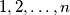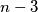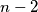### MEMO 2011 pojedinačno problem 2

Kvaliteta:
Avg: 3,0
Težina:
Avg: 6,0
Let$n \geq 3$ be an integer. John and Mary play the following game: First John labels the sides of a regular$n$-gon with the numbers$1, 2,\ldots, n$ in whatever order he wants, using each number exactly once. Then Mary divides this$n$-gon into triangles by drawing$n-3$ diagonals which do not intersect each other inside the$n$-gon. All these diagonals are labeled with number$1$. Into each of the triangles the product of the numbers on its sides is written. Let S be the sum of those$n - 2$ products.

Determine the value of$S$ if Mary wants the number$S$ to be as small as possible and John wants$S$ to be as large as possible and if they both make the best possible choices.
Izvor: Srednjoeuropska matematička olimpijada 2011, pojedinačno natjecanje, problem 2